1598148000

# Algorithms And Big O Notation In An Understandable Manner

Such scary words. It oozes math all over them…

This is probably what you’re thinking if you’re new to computer science and programming. But really, they aren’t that hard to grasp, once you understand the basics of them.

the Big-O is a way of figuring out how efficient an algorithm is. When you’re working on a big project with a huge database, making your code run as efficient as possible can save huge costs in the long run. You may ask — What is an algorithm?

### Algorithms

You can think of an algorithm as a recipe. A set of instructions on how to complete a task. Imagine getting a glass of milk. The algorithm for doing such a task might look something like this

1. Open cupboard
2. Get a glass from the cupboard
3. Close cupboard
4. Open fridge
5. Take the milk carton out of the fridge
6. Open milk carton
7. Pour milk into the glass
8. Close milk carton
9. Put milk carton back into the fridge
10. Close fridge

#algorithms #learn-programming #python #big o

## Buddha Community1598796300

## Understand Big O notation in 7 minutes

The Big O notation is a notion often completely ignored by developers. It is however a fundamental notion, more than useful and simple to understand. Contrary to popular belief, you don’t need to be a math nerd to master it. I bet you that in 7 minutes, you’ll understand everything.

#### What is the Big O notation ?

The Big O notation (or algorithm complexity) is a standard way to measure the performance of an algorithm.** It is a mathematical way of judging the effectiveness of your code.** I said the word mathematics and scared everyone away. Again, you don’t need to have a passion for math to understand and use this notation.

This notation will allow you to measure the growth rate of your algorithm in relation to the input data.** It will describe the worst possible case for the performance of your code.** Today, we are not going to talk about space complexity, but only about time complexity.

And it’s not about putting a timer before and after a function to see how long it takes.The problem is that the timer technique is anything but reliable and accurate. With a simple timer the performance of your algo will change greatly depending on many factors.

• The language you use

The Big O notation solves all these problems and allows us to have a reliable measure of the efficiency of all the code you produce. The Big O is a little name for “order of magnitude”.

#technical #understand #big o notation #big data1598508720

## What Is Big O Notation?

As programmers, we often find ourselves asking the same two questions over and over again:

1. How much time does this algorithm need to complete?
2. How much space does this algorithm need for computing?

To put it in other words, in computer programming, there are often multiple ways to solve a problem, so

1. How do we know which solution is the right one?
2. How do we compare one algorithm against another?

The big picture is that we are trying to compare how quickly the runtime of algorithms grows with respect to the size of their input. We think of the runtime of an algorithm as a function of the size of the input, where the output is how much work is required to run the algorithm.

To answer those questions, we come up with a concept called Big O notation.

• Big O describes how the time is taken, or memory is used, by a program scales with the amount of data it has to work on
• Big O notation gives us an upper bound of the complexity in the worst case, helping us to quantify performance as the input size becomes arbitrarily large
• In short, Big O notation helps us to measure the scalability of our code

### Time and Space Complexity

When talking about Big O Notation it’s important that we understand the concepts of time and space complexity, mainly because_ Big O Notation_ is a way to indicate complexities.

Complexity is an approximate measurement of how efficient (or how fast) an algorithm is and it’s associated with every algorithm we develop. This is something all developers have to be aware of. There are 2 kinds of complexities: time complexity and space complexity. Time and space complexities are approximations of how much time and space an algorithm will take to process certain inputs respectively.

Typically, there are three tiers to solve for (best case scenario, average-case scenario, and worst-case scenario) which are known as asymptotic notations. These notations allow us to answer questions such as: Does the algorithm suddenly become incredibly slow when the input size grows? Does it mostly maintain its fast run time performance as the input size increases?

#performance #development #big o complexity #big o notation #big data1598148000

## Algorithms And Big O Notation In An Understandable Manner

Such scary words. It oozes math all over them…

This is probably what you’re thinking if you’re new to computer science and programming. But really, they aren’t that hard to grasp, once you understand the basics of them.

the Big-O is a way of figuring out how efficient an algorithm is. When you’re working on a big project with a huge database, making your code run as efficient as possible can save huge costs in the long run. You may ask — What is an algorithm?

### Algorithms

You can think of an algorithm as a recipe. A set of instructions on how to complete a task. Imagine getting a glass of milk. The algorithm for doing such a task might look something like this

1. Open cupboard
2. Get a glass from the cupboard
3. Close cupboard
4. Open fridge
5. Take the milk carton out of the fridge
6. Open milk carton
7. Pour milk into the glass
8. Close milk carton
9. Put milk carton back into the fridge
10. Close fridge

#algorithms #learn-programming #python #big o1597227180

## An Overview of Big-O Notation

### How the efficiency between same purpose algorithms are judged

When you first started programming, the primary concern was figuring out an algorithm (or function, when put into practice) that would accomplish the task at hand. As your skills progressed, you started working on larger projects and studying concepts that would prepare you for a career in software engineering. One of the first concepts you would inevitably come across is asymptotic notation, or what is colloquially known as Big O Notation.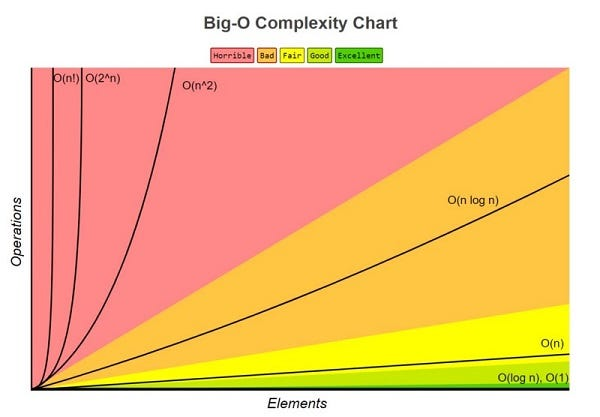In short, Big O Notation describes how long it takes a function to execute (runtime) as the size of an input becomes arbitrarily large. Big O can be represented mathematically as O(n), where “O” is growth rate (or order) of the function, and “n” is the size of the input. Translated into English, the runtime grows on the order of the input, or in the case of say O(n²), the order of the square of the size of the input.

This is a very important concept that will come up not only in your technical interviews, but also during your career when implementing solutions to handle large datasets. In this post I’ll give a brief overview on Big O analysis, simplifications, and calculations.

### Big O Analysis

When using Big O to analyze an algorithm (asymptotic analysis), it should be noted that it primarily concerns itself with the worst and average case scenarios. For example, the runtime of an algorithm which sequentially searches a data set for a value that happens to be last, would be the worst case scenario.

Figuring out the worst case scenario is safe and thus never underestimated, though sometimes it may be overly pessimistic. Ultimately whether you analyze for worst or average case will depend on the use of your algorithm. For the typical problem the average case of an algorithm may be suitable, for cryptographic problems it’s usually best to seek out the worst case.

### Heuristics for Calculating Big O

When calculating Big O, there are a couple of shortcuts that can help you

expedite the process:

• Arithmetic, assignment, accessing an element in a array/object (by index/key) are all constant time, O(1)
• In a loop, the runtime is the loop itself multiplied by whatever is in the loop

Keep these in mind for when you calculate the Big O for an algorithm I’ll provide towards the end.

#programming #big-o-notation #algorithms #algorithms1594945560

## Big O Notation and Time Complexity

Big O notation is a simplified analysis of an algorithm’s efficiency. Big O notation gives us an algorithm’s complexity in terms of input size, N. It gives us a way to abstract the efficiency of our algorithm or code from the machines/computers they run on. We don’t care how powerful our machine is, but rather, the basic steps of the code. We can use big O to analyze both time and space. I will go over how we can use Big O to measure time complexity using Ruby for examples.

Types of measurement

There are a couple of ways to look at an algorithm’s efficiency. We can examine worst-case, best-case, and average-case. When we examine big O notation, we typically look at the worst-case. This isn’t to say the other cases aren’t as important.

General rules

1. Ignore constants

5n ->O(n)

Big O notation ignores constants. For example, if you have a function that has a running time of 5n, we say that this function runs on the order of the big O of N. This is because as N gets large, the 5 no longer matters.

2. In the same way that N grows, certain terms “dominate” others

Here’s a list:

O(1) < O(logn) < O(n) < O(nlogn) < O(n²) < O(2^n) < O(n!)

We ignore low-order terms when they are dominated by high order terms.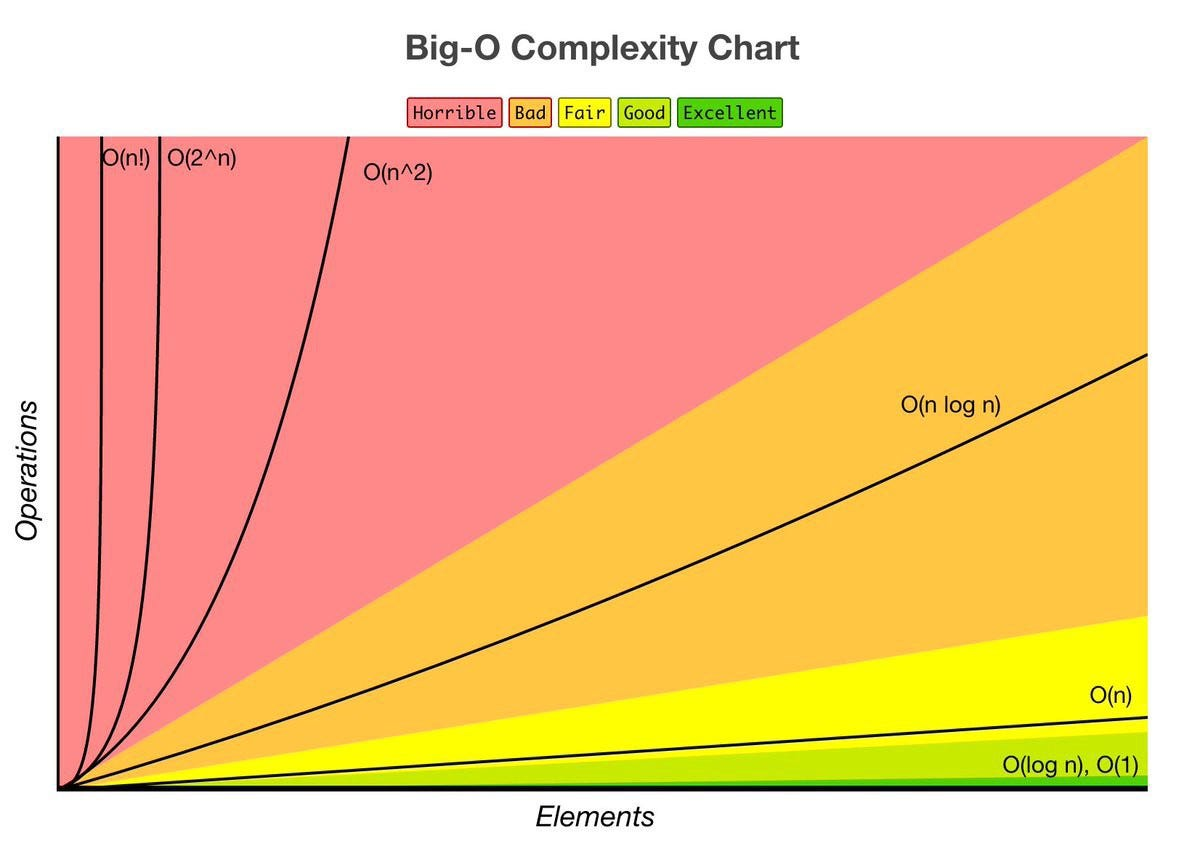Constant Time: O(1)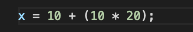This basic statement computes x and does not depend on the input size in any way. This is independent of input size, N. We say this is a “Big O of one” or constant time.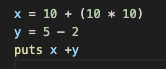total time = O(1) + O(1) + O(1) = O(1)

What happens when we have a sequence of statements? Notice that all of these are constant time. How do we compute big O for this block of code? We simply add each of their times and we get 3 * O(1). But remember we drop constants, so this is still big O of one.

Linear Time: O(n)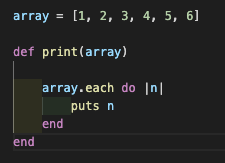The run time of this method is linear or O(n). We have a loop inside of this method which iterates over the array and outputs the element. The number of operations this loop performs will change depending on the size of the array. For example, an array of size 6 will only take 6 iterations, while an array of 18 elements would take 3 times as long. As the input size increases, so will the runtime.Big O notation is a simplified analysis of an algorithm’s efficiency. Big O notation gives us an algorithm’s complexity in terms of input size, N. It gives us a way to abstract the efficiency of our algorithm or code from the machines/computers they run on. We don’t care how powerful our machine is, but rather, the basic steps of the code. We can use big O to analyze both time and space. I will go over how we can use Big O to measure time complexity using Ruby for examples.

Types of measurement

There are a couple of ways to look at an algorithm’s efficiency. We can examine worst-case, best-case, and average-case. When we examine big O notation, we typically look at the worst-case. This isn’t to say the other cases aren’t as important.

General rules

#time-complexity #algorithms #big-o-notation #flatiron-school #algorithms